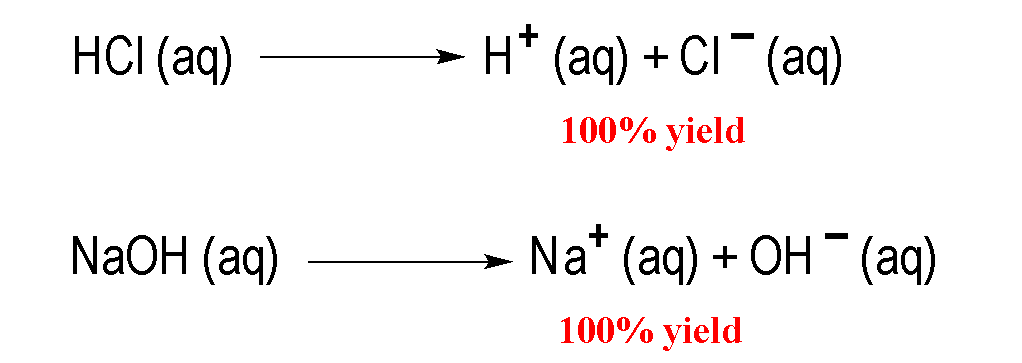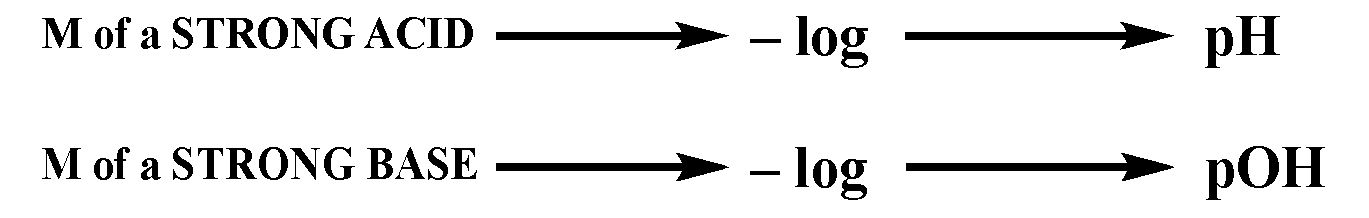Clutch Prep is now a part of Pearson
Ch.10 Acids and BasesWorksheetSee all chapters

# pH of Strong Acids & Bases

See all sections
Sections
Acid-Base Introduction
Arrhenius Acid and Base
Bronsted Lowry Acid and Base
Acid and Base Strength
Ka and Kb
The pH Scale
Auto-Ionization
pH of Strong Acids & Bases
Acid-Base Equivalents
Acid-Base Reactions
Gas Evolution Equations (Simplified)
Ionic Salts (Simplified)
Buffers
Henderson-Hasselbalch Equation
Strong Acid Strong Base Titrations (Simplified)

Concept #1: Calculating the pH of a Strong Acid or Strong Base.

Transcript

Hey guys, in this new video, we're going to take a look at the connection between pH and pOH and how they relate to strong acids and strong bases. Remember, we've talked about this before. We're going to say that strong acids and strong bases are considered to be strong electrolytes. Remember, strong electrolytes completely ionize. That means they break up a hundred percent. You may even hear that these strong acids and bases are called highly electrolytic. That also means they break up completely, highly electrolytic. If we take a look here, we have HCl and NaOH. Remember, from the rules we've learned, we know HCl is a strong binary acid and we know NaOH is a strong base because we have a group 1A ion, Na+ connected to OH-. What happens here, we have a single arrow going forward because they break up a hundred percent to give us these ions.
We'd say that the product side is highly favored. Just realize, if we have a strong acid or a strong base, I’ve said it before, we don't have to use an ICE chart in order to find pH or pOH. If you have a strong acid, take the negative log to find pH. If you have a strong base, take the negative log to find pOH. Let's take a look at example 1.Example #1: Calculate the pH of a 0.0782 M solution of CaH2.

Transcript

In example 1, it says: Calculate the pH of a 0.0782 molar solution of calcium hydride. We know that calcium hydride is a strong base, because remember, we have calcium which is Ca2+ connecting with H-, our hydride ion. What happens here? The two from here came here, the one from here came here. That's how we got calcium hydride. What we should realize here is that there are two calcium hydrides in the formula. Now, this is what you need to remember when it comes to strong bases. For strong bases, you have to be very careful. We're going to say that this is not the real concentration of our strong base. You're going to say that when you have strong bases, you have to look at the number of OH-, H-, NH2-, O2-. You have to look at to see how many of each you have in order to determine the real concentration of that strong base. In this particular question, we have two H-. Because we have two of them, we have to multiply this by two. That will give us the correct concentration of our strong base. You have to remember that.
For example, if I had COH2 and there was 0.100 molar of it, again because you have two OHs, you’d have to multiply that by two. If you had calcium amide, you would have two NH2, so again you’d have to multiply the concentration times two to get the correct concentration. Now what we're going to do is we're going to multiply the initial concentration times two to get the real concentration. When we do that, we're going to get our correct concentration. That's the number that we're going to plug in. When we do that, and I'm going to remove myself guys from the image so we have more room to work with. When we do that, we’re going to get a new number, a new value for our OH- concentration.
Here we're going to get 0.1564 molar. That becomes our new concentration. We're going to say pOH equals negative log of OH-. It becomes negative log of 0.1564 molar. Some of you may be asking, “But Jules, this is saying the concentration of OH-. We don't have OH-. We have H-. How do they relate?” Just realized that all four of these ions can be treated as being the same. They all feed into the concentration of OH-. OH- is just understood as a general term for these strong basic ion of a strong base. If I have two OH-, that's equivalent to two H-, equivalent to two NH2-. The same thing. When we take the negative log of this, this will give us our pOH. It’s going to give us 0.814 for our pOH.
But remember, let's pay attention. I didn't ask for the pOH, I asked for the pH. We need to use the next equation, pH plus pOH equals 14. Plug in what we know for pOH, 0.81. We need pH so subtract 0.81 from both sides. So pH here equals 13.19. So that will be our pH for this first question.
Hopefully you guys didn't get lost but what I was saying. Just remember, for strong bases you have to look to see how many OH- you have, H- you have, NH2- you have and O2- you have. Their number determines the correct concentration of our strong base. Right now, what you need to realize is we only do this for strong base at this point. If we had a strong acid such as H2SO4, that strong acid has two acidic Hs. But we wouldn't need to multiply the concentration times two because when it comes to acids, the first H coming off is extremely strong. But the second one that comes off is extremely weak. Because the second H+ is extremely weak, we wouldn't need to multiply the concentration times two. We only do this multiplication of concentrations when we have strong bases. Later on when we deal with acid-base titrations and buffers, we'll talk about how acids play a role.Example #2: Calculate the pH of a 0.000550 M HBr solution to the correct number of significant figures.

Practice: Calculate the pH of 50.00 mL of 4.3 x 10-7 M H2SO4.